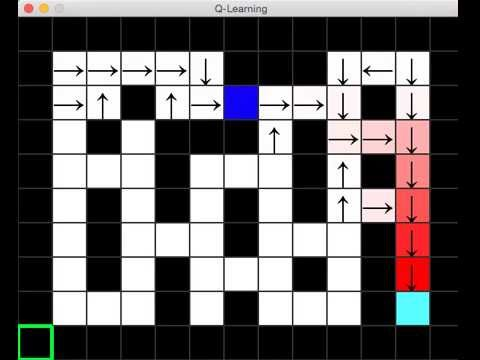# Introduction

This time, I would like to explore the maze using reinforcement learning, especially Q-learning.

# Q learning

## Overview

To put it simply, a value called Q value is retained for each pair of "state" and "behavior", and the Q value is updated using "reward" or the like. Actions that are more likely to get a positive reward will converge to a higher Q value. In the maze, the squares in the passage correspond to the state, and moving up, down, left, and right corresponds to the action. In other words, it is necessary to keep the Q value in the memory for the number of squares in the passage * the number of action patterns (4 for up, down, left, and right). Therefore, it cannot be easily adapted when there are many "state" and "action" pairs, that is, when the state and action space explodes.

This time, we will deal with the problem that the number of squares in the aisle is 60 and the number of actions that can be taken is four, about 240 in the vertical and horizontal directions.

## algorithm

### Update Q value

Initially, all Q values are initialized to 0. The Q value is updated every time the action $a$ is taken in the state $s_t$.

Q(s_t, a) \leftarrow Q(s_t, a) + \alpha(r_{t+1} + \gamma \max_{p}{Q(s_{t+1}, p)} -Q(s_t, a))

### Action selection

This time we will use ε-greedy. Random actions are selected with a small probability of ε, and actions with the maximum Q value are selected with a probability of 1-ε.

# Source code

The code has been uploaded to Github. Do it as python map.py. I wrote it about two years ago, but it's pretty terrible.

# Experiment

## environment

The experimental environment is as shown in the photo below. The light blue square in the lower right is the goal, the square in the upper left is the start, and the blue squares are the learning agents. When you reach the goal, you will receive a positive reward. Also, the black part is the wall and the agent cannot enter. So the agent has no choice but to go through the white passage. The Q value of each cell is initialized to 0, but when the Q value becomes larger than 0, the largest Q value of the four Q values in that cell is the shade of color, and the action is displayed by an arrow. It is a mechanism.## result

The experimental results are posted on youtube. You can see that the Q value is propagated as the agent reaches the goal.# in conclusion

I want to try Q-learning + neural network